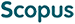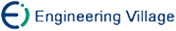王万森, 何华灿. 基于泛逻辑学的概率命题逻辑的研究与分析[J]. 计算机研究与发展, 2005, 42(7): 1204-1209.
 引用本文: 王万森, 何华灿. 基于泛逻辑学的概率命题逻辑的研究与分析[J]. 计算机研究与发展, 2005, 42(7): 1204-1209.Wang Wansen, He Huacan. Research and Analysis of Probability Logic Based on Universal Logics[J]. Journal of Computer Research and Development, 2005, 42(7): 1204-1209.
 Citation: Wang Wansen, He Huacan. Research and Analysis of Probability Logic Based on Universal Logics[J]. Journal of Computer Research and Development, 2005, 42(7): 1204-1209.## Research and Analysis of Probability Logic Based on Universal Logics

• 摘要: 概率逻辑是不确定推理的一个重要逻辑基础，但其目前还不太完善.泛逻辑学是何华灿教授在探索各种不确定性问题求解中建立起来的一种新的柔性逻辑体系.理论上，概率逻辑仅是泛逻辑学的一个特例.在对目前比较典型的几种概率逻辑模型进行分析的基础上，基于命题泛逻辑学的思想和方法，指出了概率命题逻辑中存在的一些主要问题，探讨了解决这些问题的思路与方法.

Abstract: Probability logic is an important foundation for uncertainty reasoning. However, there is a lot more to do to make it perfect. Universal logics is a new flexible logic system established in the study of various nondeterministic problems. It is an abstraction for building concrete logic systems. Theoretically, probability logic is a special example of universal logics. In this paper, several classical models are analyzed, problems in these models are pointed out, and new methods to solve them based on universal logics are presented./下载:  全尺寸图片 幻灯片
• 分享
• 用微信扫码二维码

分享至好友和朋友圈